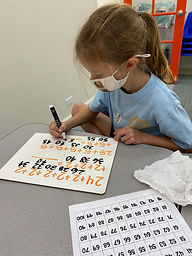Ms. Brianne

Target 1​

Lesson Type:

New

Number Operation

:

Number System

Skip count by twos and fives up to 100.

1:

Connect skip counting to repeated addition and subtraction.

2:

Explore the counting patterns through skip counting by twos from zero to 100.

3:

Explore the counting patterns through skip counting by fives from zero to 100.

3rd

Vocabulary:

Skip Count, Sequence, Pattern

Activities:

• Students identified the numbers for skip counting by 5's and 2's on a large hundred chart.
• Students noticed counting patterns in skip counting by 5's and 2's.
• Students used skip counting to complete repeated addition equations.
• Students related skip counting by 5's to counting nickels.
• Students completed a picture maze following the sequence of skip counting by 2's.Home Exploration

Guiding Questions:Absent Students:

Target 2

:

1:

Understand the breakdown of hours into minutes.

2:

Recognize that a time on the hour will have the minute hand on the 12 on an analog clock and 00 on the digital clock.

3:

Recognize that a time on the half-hour will have the minute hand on the 6 on an analog clock and 30 on the digital clock.

4:

Apply knowledge of skip counting by fives to read an analog clock.

2nd

Vocabulary:

Analog, Digital, Hour, Minute

Activities:

• Students used the analog clock to read and show times in 5 minute increments.
• Students practiced skip counting by 5's to read an analog clock.
• Students understood there are 5 minutes between each number shown on the clock.
• Students wrote the digital time when shown an analog clock.
• Students played a game where they rolled a dice and had to read the time on a clock with the matching number.Home Exploration

Guiding Questions:Target 3

:

Vocabulary:

Activities:Home Exploration